## Simple Harmonic Motion

|SHM | Wave terms | Wave Phenomena |

associated with Comp 10.1 / chap 13

HW problems; answers: 1-4 and book

 SHM Harmonic motion - presence of a restoring force Simple Harmonic motion: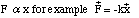a linear restoring force, the force is directly proportional to the displacement and in the opposite direction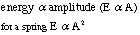vocabulary frequency/period/angular frequency oscillation, cycle, vibration, equilibrium (rest) position Amplitude (linear/angular) axis of symmetry simple pendulum equations/limitations Resonance damping Mode: -transverse -longitudinal (translational) rarefaction/compression -torsional (rotational) period (T) in sec - the time it takes for one complete oscillation, vibration, or cycle frequency (f) =1/T in Hz (s^-1) - the number of oscillations, vibrations, or cycles per second angular frequency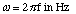cycle/oscillation/vibration: one complete motion tothe point where the motion starts to repeat amplitude (A) typically in m or °/rad - the maximum displacement of the object from its equilibrium position or its axis of symmetry 3 types of SHM: 1) longitudinal 2) transverse 3) torsional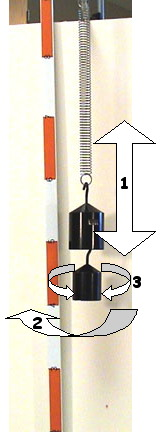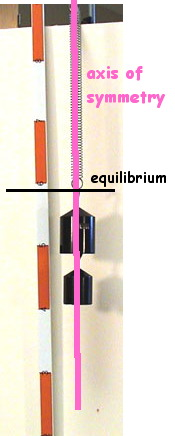Longitudinal: the mass is displaced parallel (or antiparallel) to the axis of symmetry or the amplitude is along the axis of symmetry creating areas of compression and rarefaction; amplitude is measured as a distance transverse: the mass is displaced perpendicular to the axis of symmetry; amplitude is measured as a distance or an angle (angle is preferred) torsional: the mass is rotated around the axis of symmetry; amplitude is measured as an angle simple pendulum: system where the mass is concentrated a distance L from the pivot point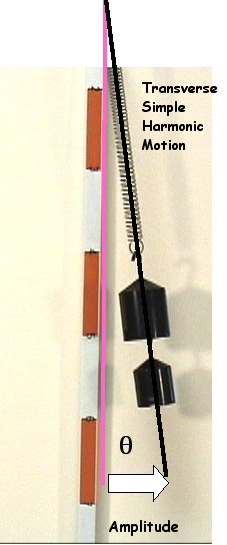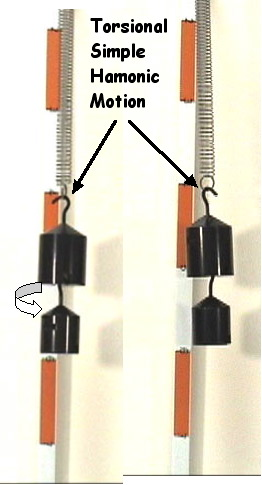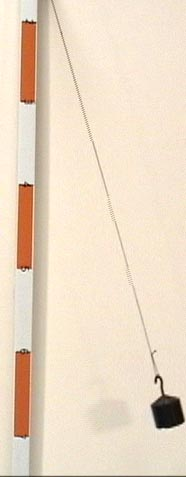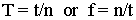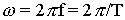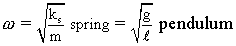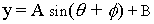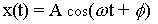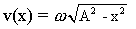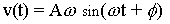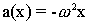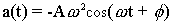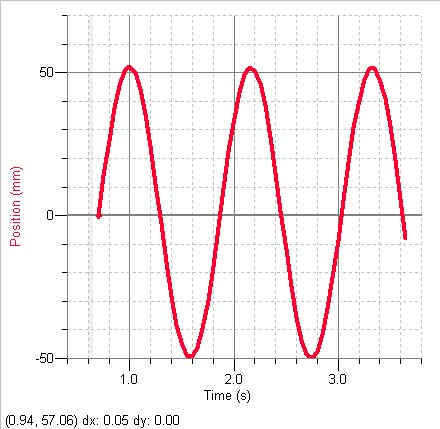distance vs time What is the period?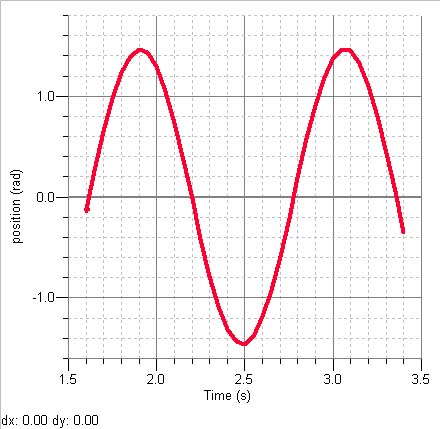angle vs time What is the period?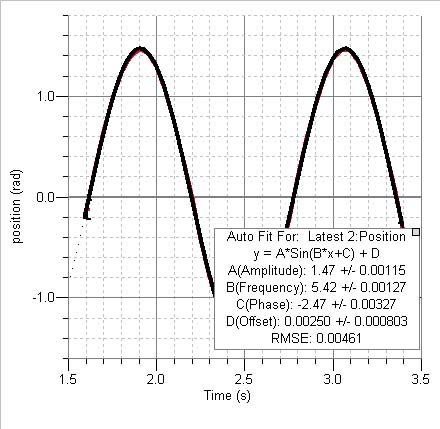What is the actual equation (with units) for the curve? The amplitude is mesured in ___. The value "B" really represents ____. Calculate the period of the motion.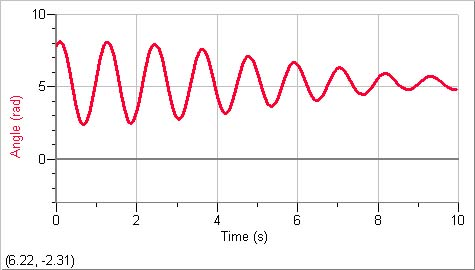damped oscillations Amplitude decreases caused by an outside force. resonance: when two oscillating systems share a common (or multiple of the same) frequency